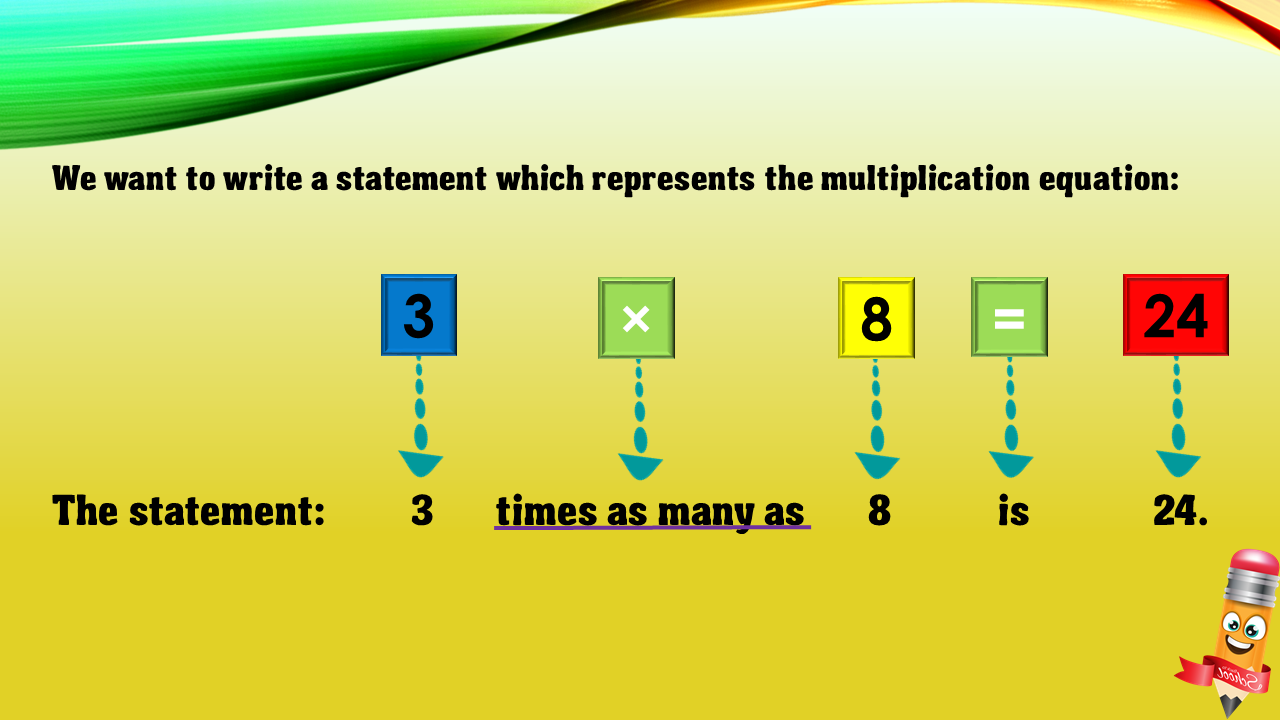1
visibility

Choose one statement which represents the equation:

3 × 8 = 24 ?

• A

8 times as many as 24 is 3.

• B

3 more than 8 is 24.

• C

8 less than 24 is 3.

• D

3 times as many as 8 is 24.